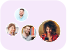Product Edition:3rd Edition
Author: Morris W. Hirsch
Book Name: Differential Equations, Dynamical Systems, and an Introduction to Chaos
Subject Name: Maths

# Differential Equations, Dynamical Systems, and an Introduction to Chaos 3rd Edition Solutions

0 out of 5.0
45 reviews45 Students
have requested for homework help from this book

Hirsch, Devaney, and Smaleâ€™s classic Differential Equations, Dynamical Systems, and an Introduction to Chaos has been used by professors as the primary text for undergraduate and graduate level courses covering differential equations. It provides a theoretical approach to dynamical systems and chaos written for a diverse student population among the fields of mathematics, science, and engineering. Prominent experts provide everything students need to know about dynamical systems as students seek to develop sufficient mathematical skills to analyze the types of differential equations that arise in their area of study. The authors provide rigorous exercises and examples clearly and easily by slowly introducing linear systems of differential equations. Calculus is required as specialized advanced topics not usually found in elementary differential equations courses are included, such as exploring the world of discrete dynamical systems and describing chaotic systems.

5
23
4
9
3
4
2
7
1
2

0

## Students who viewed this book also checked out